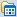MJ-majun

posts - 111, comments - 1, trackbacks - 0, articles - 84
博客园 :: 首页 :: 新随笔 :: 联系 :: 订阅:: 管理公告

• zip()
#zip 拉链方法 一一对应  只要是序列类型的都可以
print(list(zip(('a','b','c'),(1,2,3))))
结果： [('a', 1), ('b', 2), ('c', 3)]

p ={'name':'xiaoma','age':18,'ciry':'shanghai'}
print(list(zip(p.keys(),p.values())))
结果：[('name', 'xiaoma'), ('age', 18), ('ciry', 'shanghai')]
• print(all(·····)) 全部对才返回 True
• print(any(···)) 只要一个是对的就返回 True
• bool
• print(bool('')) 空字符串 返回False
• print(bool(0)) 数字 0 返回False
• print(bool(None)) Nane 返回False
• 其余都是返回True• print(bytes()) 转换字节
编码转化成二进制
name = '你好'
print(bytes(name,encoding='utf-8'))

结果： b'\xe4\xbd\xa0\xe5\xa5\xbd'

#解码 （用什么编码就用什么解码）
name = '你好'
print(bytes(name,encoding='utf-8').decode('utf-8'))

结果：  你好
name = '你好'
print(bytes(name,encoding='gbk'))
print(bytes(name,encoding='gbk').decode('gbk'))

结果： b'\xc4\xe3\xba\xc3'
结果： 你好

#ascii不能编码中文
name = '你好'
print(bytes(name,encoding='ascii'))
error
• 　　print(chr(···)) 对应的ASCII码
• 　　print(dir(···)) 打印某一个对象下的各种方法
• 　　print(divmod(···)) 取商得余数 （例如做分页功能）
••• evel(····) 把字符串中的表达式进行运算
•• hash() 可hash的数据类型即不可变数据类型，不可hash的数据类型即可变数据类型
• print(help(···)) 　　查看所有的帮助
• pirnt(bin(10)) 　　10进制转2进制
• pirnt(hex(12)) 　　10进制转12进制
• pirnt(oct(12)) 　　10进制转8进制
• print(globals(···)) 　　打印全局变量
• print(locals(···)) 　　打印局部变量
• print(max(···)) 　　打印最大值
• print(min(···)) 　　打印最小值